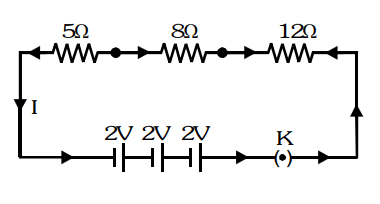# Draw a schematic diagram of a circuit consisting of a battery

Question.
Draw a schematic diagram of a circuit consisting of a battery of three cells of $2 \mathrm{~V}$ each, a 5 ohm resistor, an $8 \mathrm{ohm}$ resistor, and a $12 \mathrm{ohm}$ resistor, and a plug key, all connected in series.

solution: